Скачать презентацию W eek 2 Symmetric Cipher Model Altayeva Aigerim

lec2_security_class.ppt

• Размер: 821.3 Кб
• Количество слайдов: 23

## Описание презентации W eek 2 Symmetric Cipher Model Altayeva Aigerim по слайдам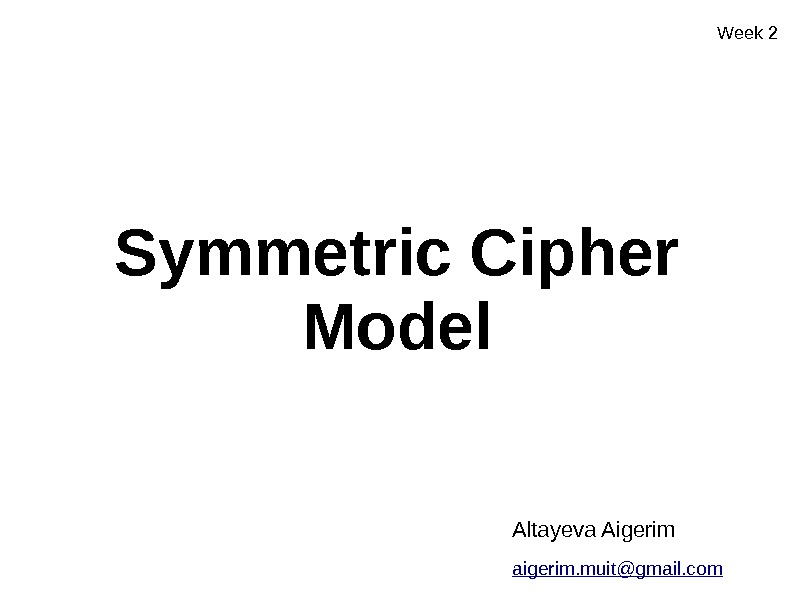W eek 2 Symmetric Cipher Model Altayeva Aigerim aigerim. [email protected] com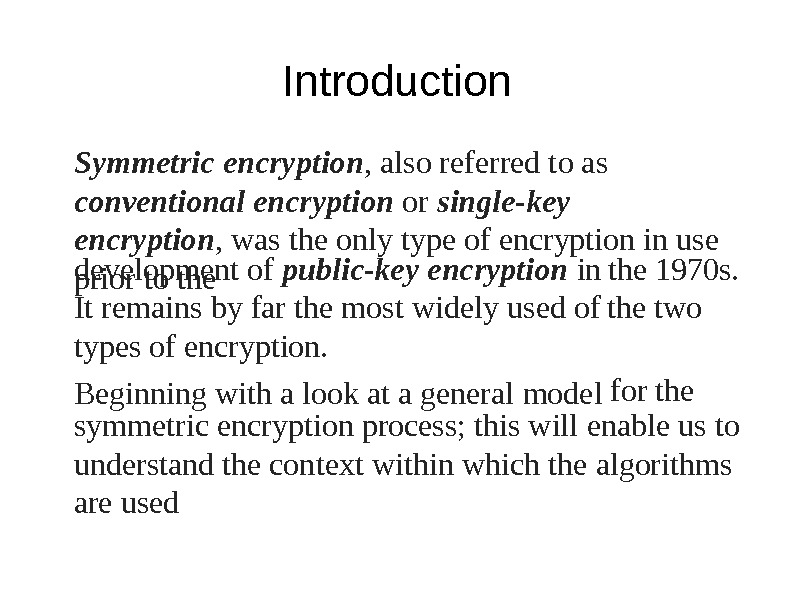Introduction Symmet r ic encryptio n , al s o re f erred t o as conventional encryption or s ingle-key encryptio n , was the only t ype of encryption i n u se prior to thedevelopment of public-key encryption in It remains by far the m o s t widely used of types of encryption. Beg i nning with a look at a general model the 197 0 s. two for the s y m metric encrypt i on process; this will en a b l e us to under s tand the con t e xt within which the algorith m s are u s ed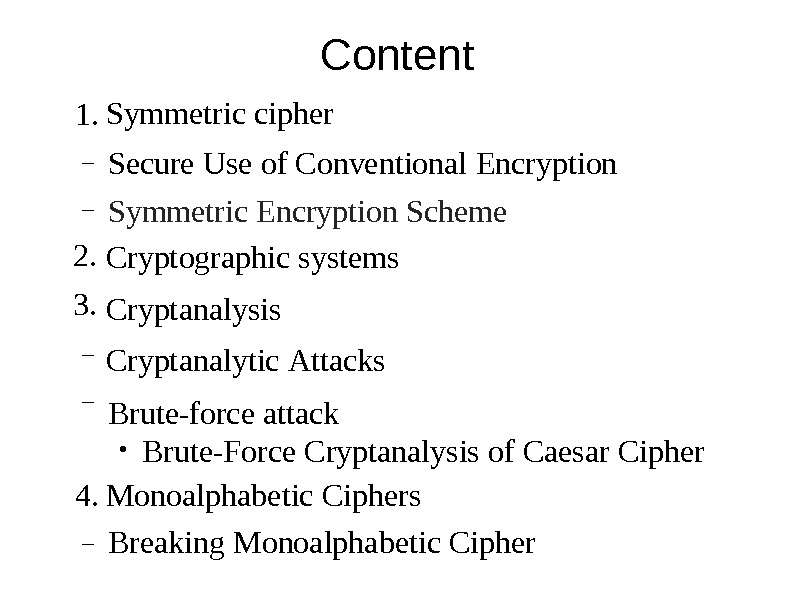Content Symmetric cipher Secure Use of Conventional Encryption 1. – – 2. 3. – – Symmetric Encryption Cryptographic s y s tems Cryptanaly s is Cryptanalytic Attacks Brute-force attack Scheme Brute-Force Cryptanaly s is of Caesar Cipher● 4. – M onoalphabetic Ciphers Breaking M onoalphabetic Cipher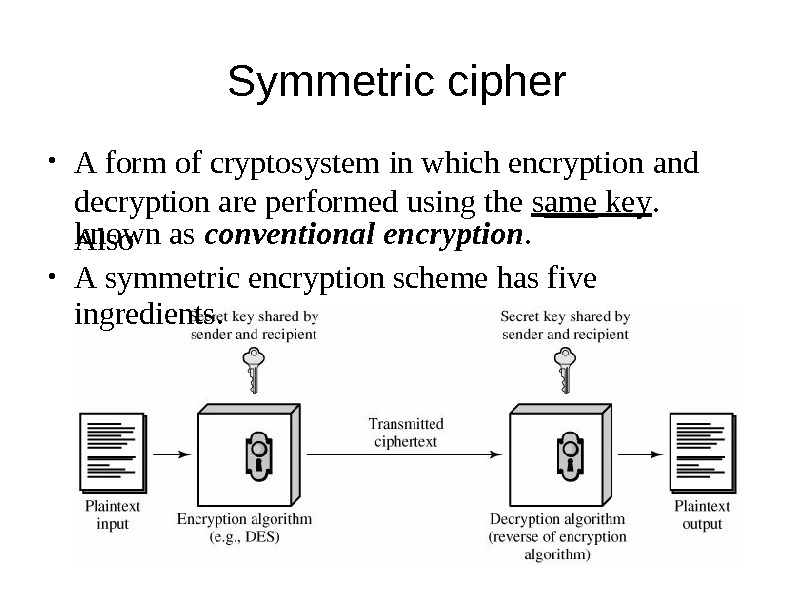Sy m metric cipher A form of cryptosystem in which encryption and decryption are performed u s ing the s ame ke y. A l s o● known as conventional encryptio n. A s ymmetric encryption s cheme has five ingredient s. ●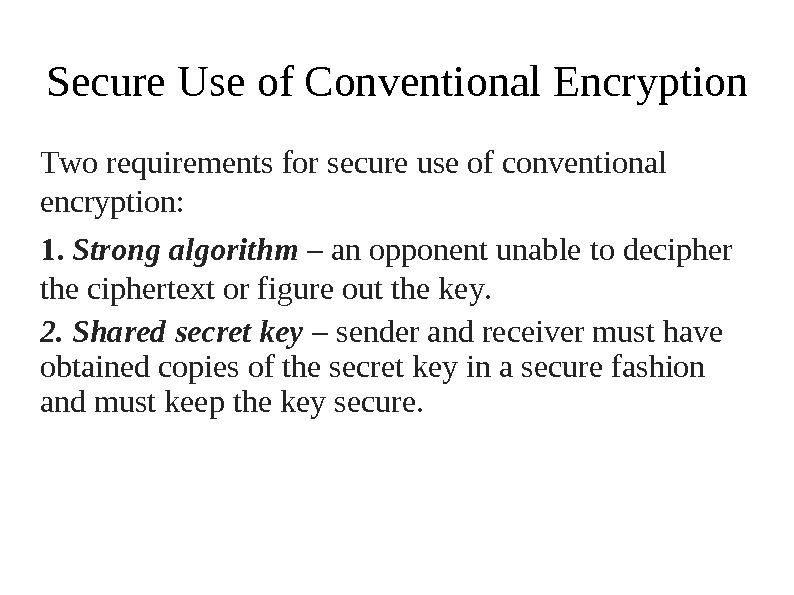Secure Use of Conventional Encryption T wo requi r e m e n t s for s ecure use of conventional encryption: 1. Strong algorithm – an opponent unable to decipher the ciphertext or figure out the ke y. 2. Shared s ecret key – s ender and r e ceiver must have obtained copies of the secret key in a secure fa s hion and must keep the key s ecure.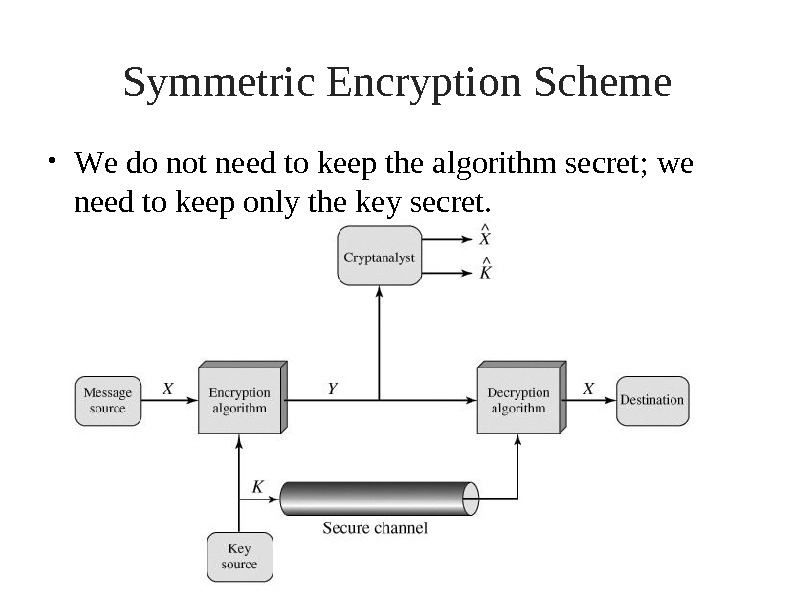Symmetric Encryption Scheme W e do n ot ne e d t o k e ep t he a l gorithm secret; we need to keep only the key s ecret. ●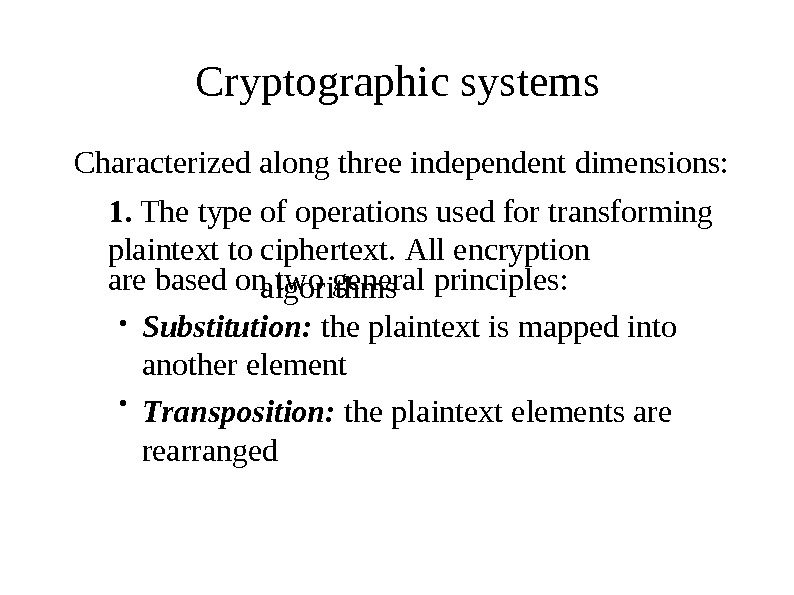Cryptographic systems Characterized 1. T h e type plaintext to along three independent dimensions: of ope r ations used for t r ansforming ciphe r text. All enc r yption algorith m sare based on two general principle s : Substitution: the plaintext is mapped into another element T r anspo s ition: the p l a i ntext elements are rearranged● ●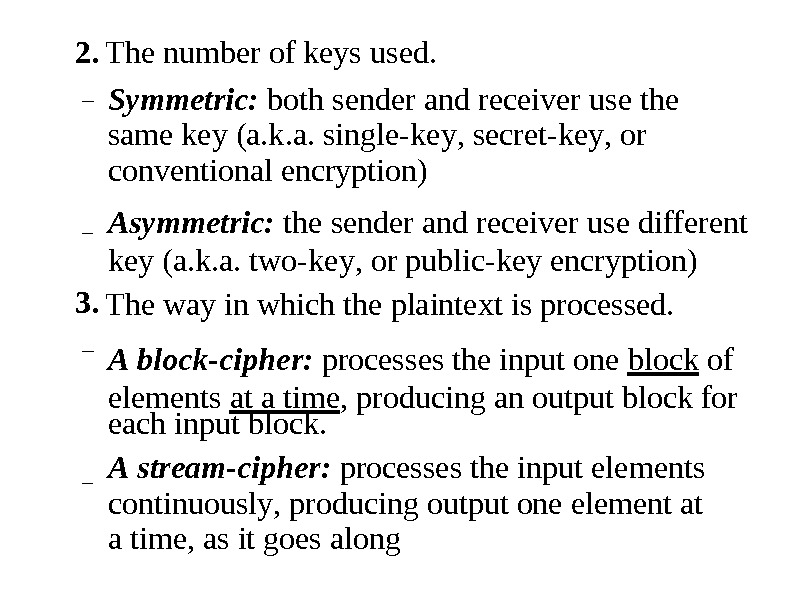2. – The number of keys u s ed. Symmet r ic: both sender and rece i ver u s e t he same key (a. k. a. single-ke y , s ec r e t -ke y , or conventional encryption) Asymmet r ic: the sender and receiver use di f fe r ent key (a. k. a. two-ke y , or public-key encryption) The way in which the plaintext is proces s ed. A block-ciphe r : proces s es the input one block of elements at a tim e , pr o ducing an output block for– 3. – each input block. A s tream-ciphe r : processes the input ele m e n t s continuou s l y , producing output one element at a time, as it goes along–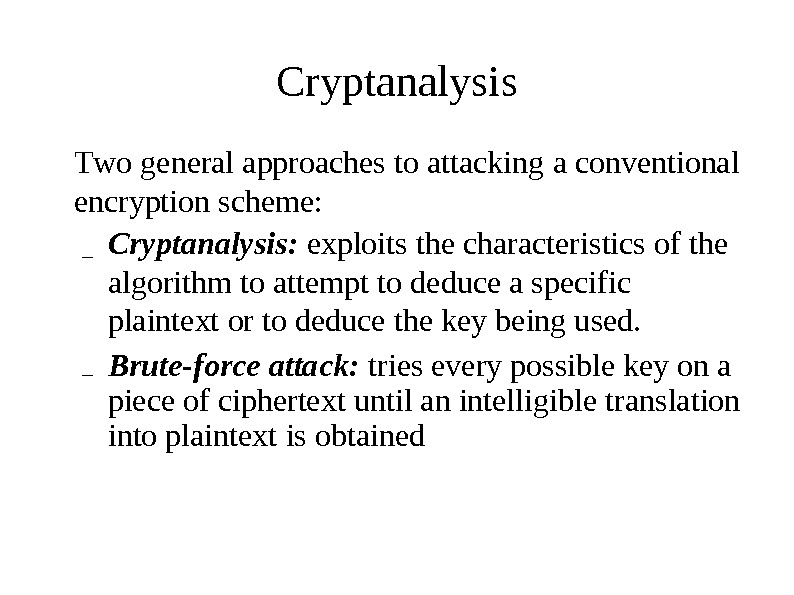Cryptanalysis T wo general approaches to attacking a conventional encryption s cheme: Cryptanaly s is: exploits the character i s tics of the algor i thm to attempt to deduce a s peci f ic plaintext or to deduce the key being u s ed. Brute-force attack: tr i es every pos s ible key on a piece of ciphe r text until an intelligible tran s lation into plaintext is obtained– –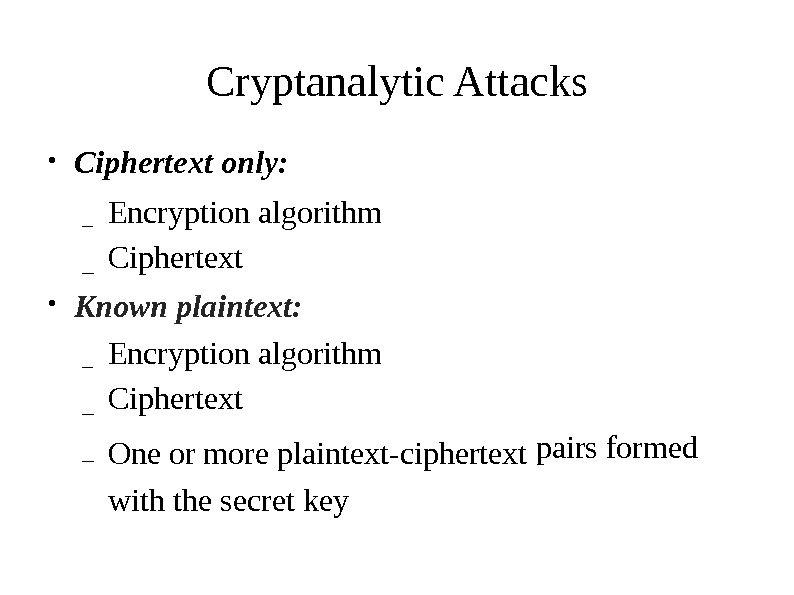Cryptanalytic Attacks Ciphertext only: ● Encryption algorithm Ciphertext– – Known plaintext: ● Encryption algorithm Ciphertext One or more plaintext-ciphertext with the s ecret key– – – pairs formed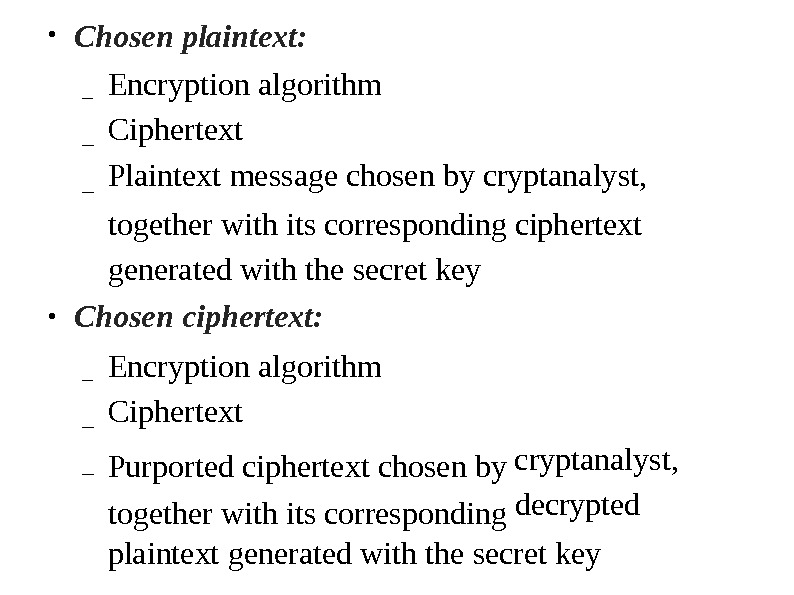Chosen plaintext: ● Encryption algorithm Ciphertext Plaintext mes s age chosen by cryptanaly s t, – – – together with its corresponding generated with the s ecret key Chosen ciphertext: ciphertext ● Encryption algorithm Ciphertext Purported ciphertext chosen by together with its corresponding– – – cryptanaly s t, decrypted plaintext generated with the s ecret key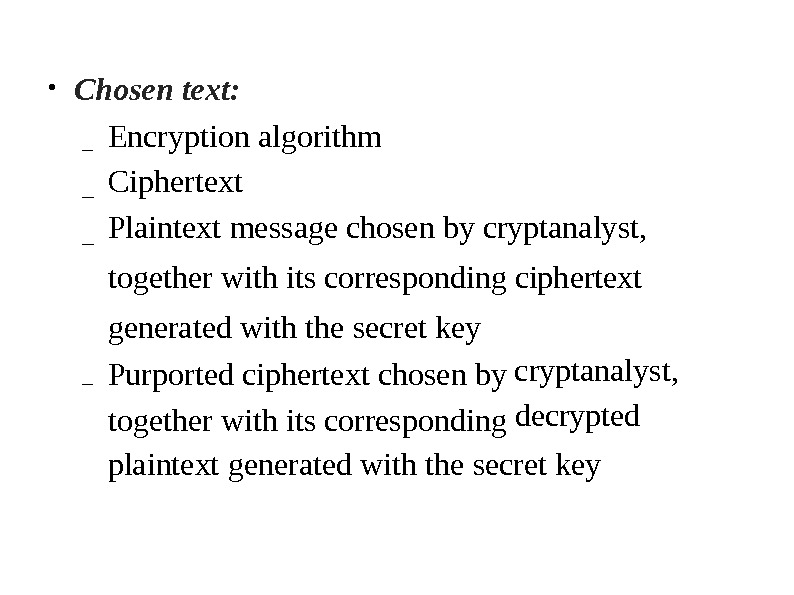Chosen text: ● Encryption algorithm Ciphertext Plaintext mes s age chosen by cryptanaly s t, – – – together with its corresponding generated with the s ecret key Purported ciphertext chosen by together with its corresponding ciphertext cryptanaly s t, decrypted– plaintext generated with the s ecret key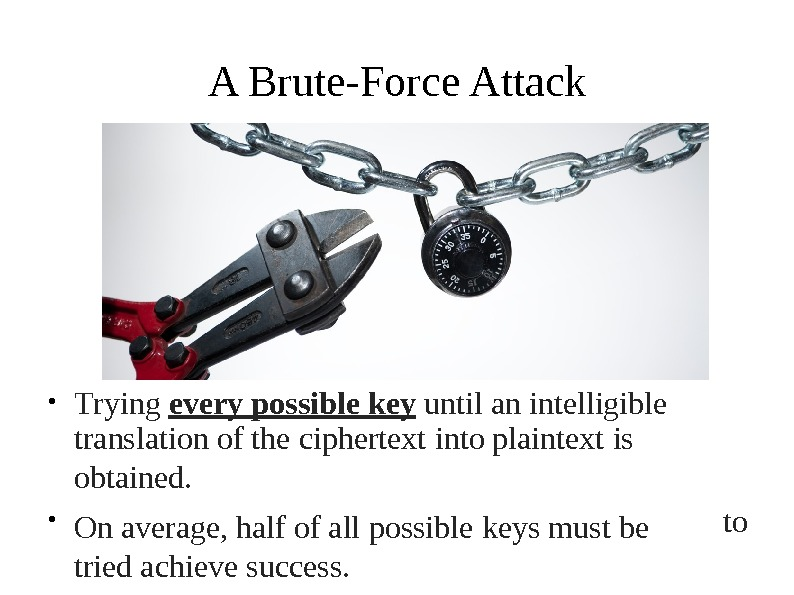A Brute-Force Attack T rying every pos s ible key until an intelligible● t r a nslation of the ciphertext into plaintext i s obtained. On average, half of all po s sible keys mu s t be t ried achieve s ucces s. to●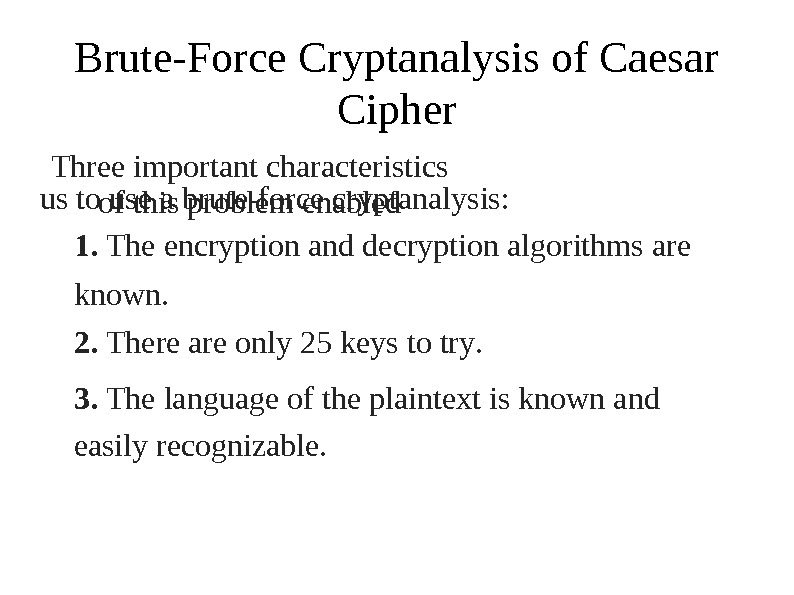Brute-Fo r ce Cryptana l ysis of Caesar Cipher Three impor t a nt ch a racteri s tics of this problem enabledus to u s e a brute-force cryptanaly s is: 1. The encryption and decryption algorithms are known. 2. There are only 25 keys to tr y. 3. The language of the plaintext is known and easily recognizable.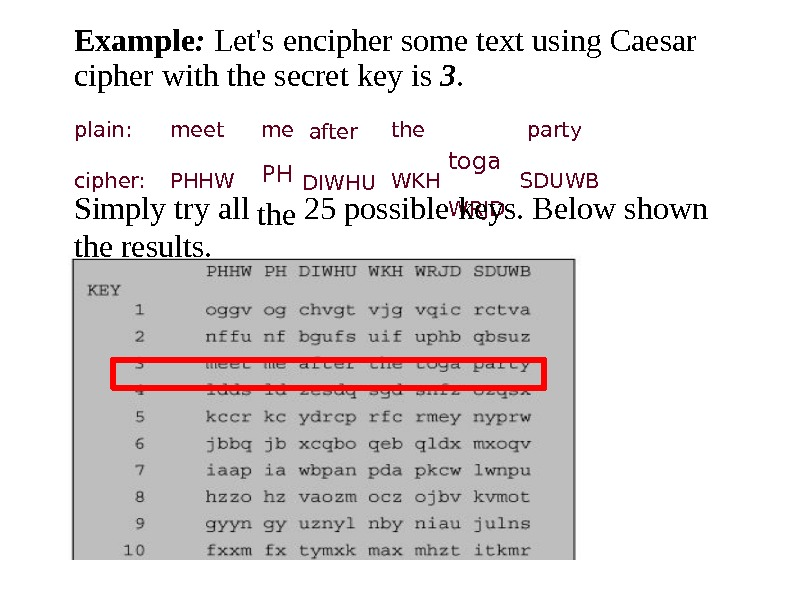Exampl e : L et ‘ s encipher some t e xt using C ae s ar cipher with the s ecret key is 3. toga WRJDplain: cipher: meet PHHW me PH the after DIWHU the WKH party SDUWB Simply t r y all the results. 25 po s sible keys. Below s hown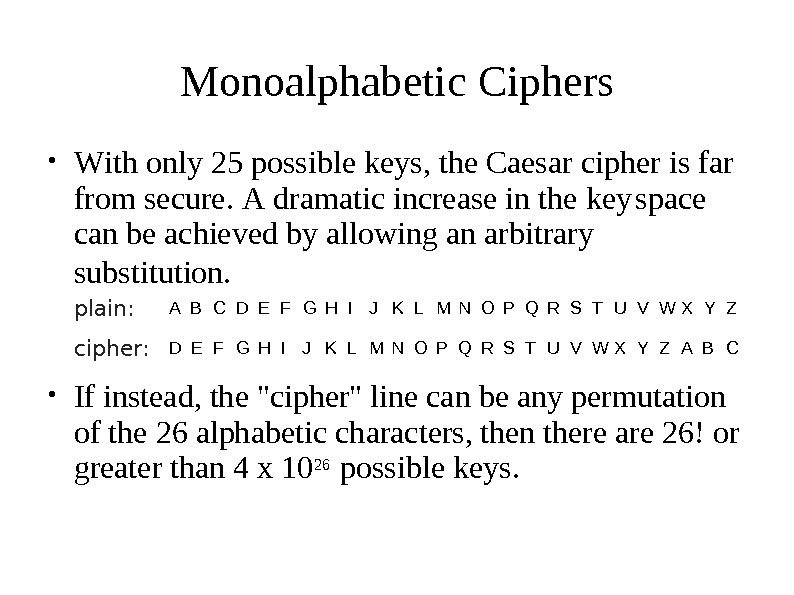Monoalphabetic Ciphers W ith only 25 po s sible keys, the Caesar cipher is far● from s ecu r e. A drama t ic increa s e in the key s pace can be achieved by s ub s titution. allowing an arbit r ary plain: cipher: A B C D E F G H I J K L M N O P Q R S T U V W X Y Z D E F G H I J K L M N O P Q R S T U V W X Y Z A B C If instead, the «cipher» line can be any pe r mutation● of the 26 alphabetic cha r acter s , then t here are 26! or greater than 4 x 1 0 26 po ss ible keys.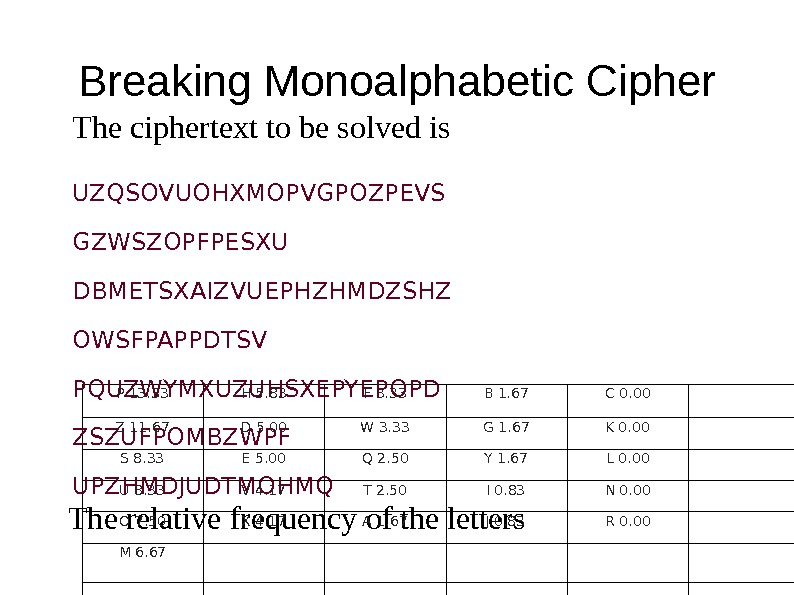Breaking Monoalphabetic Cipher The ciphertext to be s olved is UZQSOVUOHXMOPVGPOZPEVS GZWSZOPFPESXU DBMETSXAIZVUEPHZHMDZSHZ OWSFPAPPDTSV PQUZWYMXUZUHSXEPYEPOPD ZSZUFPOMBZWPF UPZHMDJUDTMOHMQ The relative frequency of the letters. P 13. 33 H 5. 83 F 3. 33 B 1. 67 C 0. 00 Z 11. 67 D 5. 00 W 3. 33 G 1. 67 K 0. 00 S 8. 33 E 5. 00 Q 2. 50 Y 1. 67 L 0. 00 U 8. 33 V 4. 17 T 2. 50 I 0. 83 N 0. 00 O 7. 50 X 4. 17 A 1. 67 J 0. 83 R 0. 00 M 6.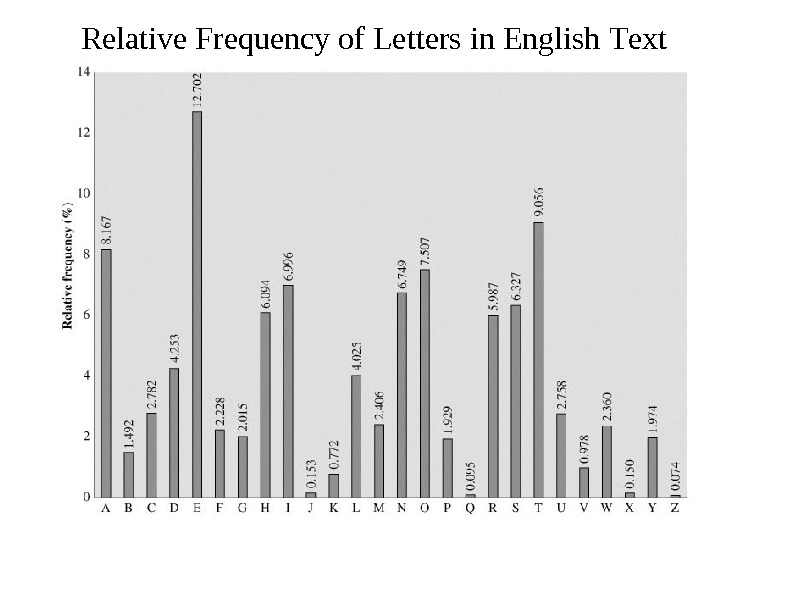Relative Frequency of Letters in English T ext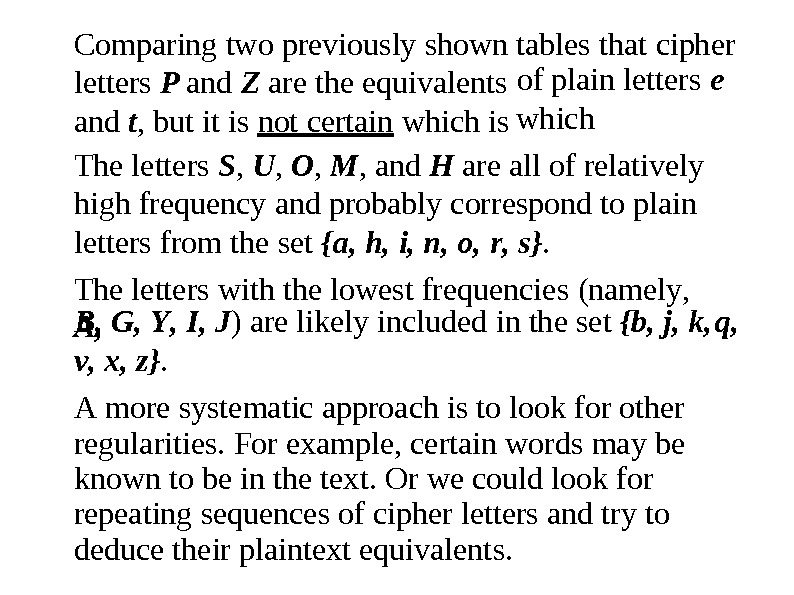Comparing two previously s hown letters P and Z are the equivalents and t , but it is not certain which is tables that cipher of plain letters which e The letters S , U , O , M , and H are all of relatively high frequency and probably cor r espond to plain letters from the s et {a, h, i, n, o, r , s}. The letters with the lowest frequencies (namel y , A, B, G, Y , I , J ) are likely included in the s et {b, j, k, v , x, z }. A mo r e s ystematic approach is to look for other regula r itie s. For ex a m ple, ce r tain words may be known to be in t he tex t. Or w e could look for repeating sequences of cipher letters and t ry to deduce their plaintext equivalent s. q,A p o we r ful tool is to look at the frequency of two-le t ter combination s , known as digrams. The most common dig r am in cipher is Z W , which appears three time s. So we make the correspondence: Z → t and W → h.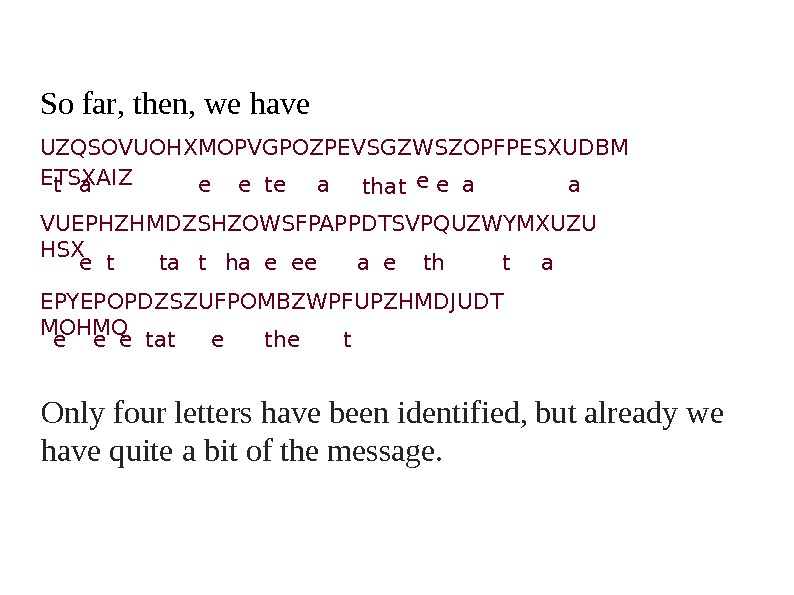So fa r , then, we have UZQSOVUOHXMOPVGPOZPEVSGZWSZOPFPESXUDBM ETSXAIZ t a e e te a that e e a a VUEPHZHMDZSHZOWSFPAPPDTSVPQUZWYMXUZU HSX e t ta t ha e ee a e th t a EPYEPOPDZSZUFPOMBZWPFUPZHMDJUDT MOHMQ e e e tat e the t Only four lette r s have quite a bit of the been identi f ied, mes s age. but already we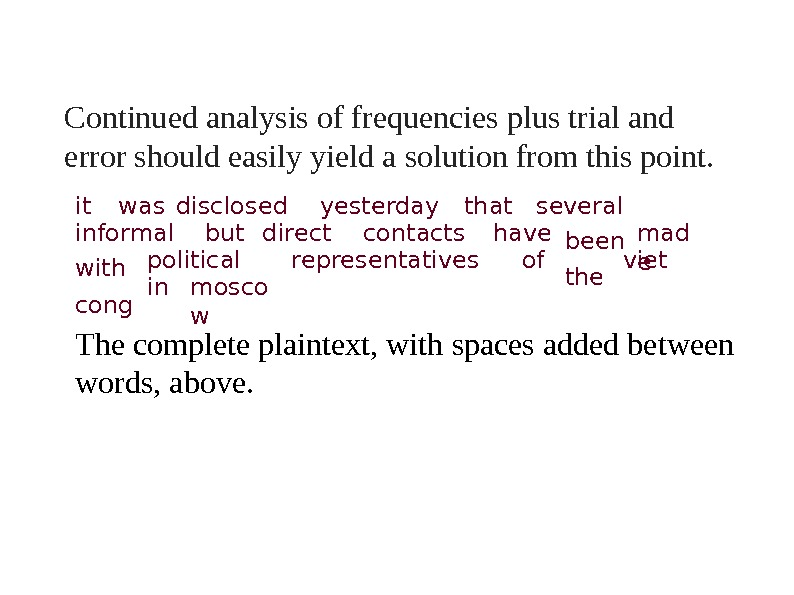Continued a n a l y s is of frequencies plus t rial and error s hould easily yield a s olution from this point. it w a s disclos e d yest e rday that seve r al inform a l but dir e ct contacts have b een t he mad e with cong political r epresentativ e s of viet in mosco w T h e comple t e p l a i ntext, with s paces added between words, above.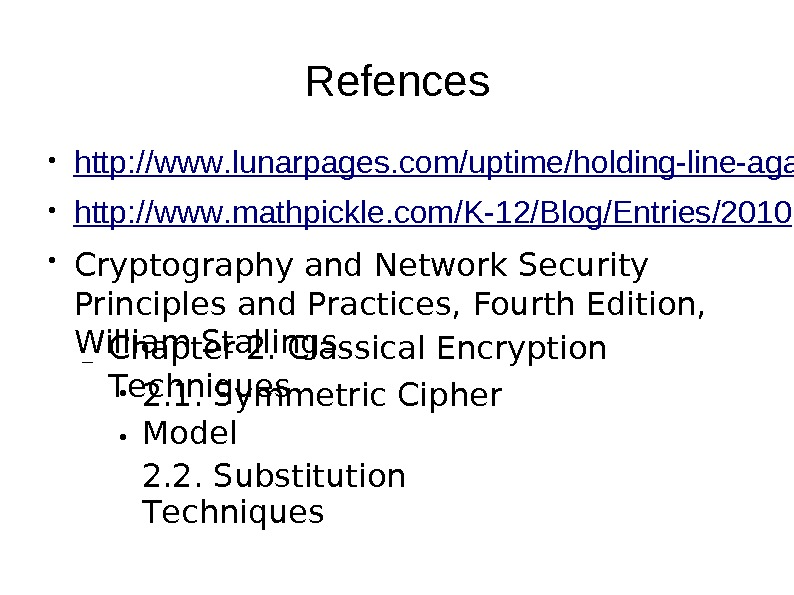Refences http: //ww w. lunarpages. com/uptime/holding-line-aga● http: //ww w. mathpickle. com/K-12/Blog/Entries/2010 Cryptog r aphy and Network Security Principles and Practices, Fourth Edition, William Stallings● ● Chapter 2. Classical Encryption T echniques– 2. 1. Symmetric Cipher Model 2. 2. Substitution T echniques● ●